手机版(适合小屏用户) 爱查股网 www.aichagu.com 股票查询最好股票网 个股数据查询好股网 关闭本站广告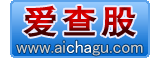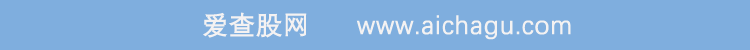# 尾盘今买明卖“一日游”选股指标，通达信筹码进出副图指标公式

2020/7/27 15:04:00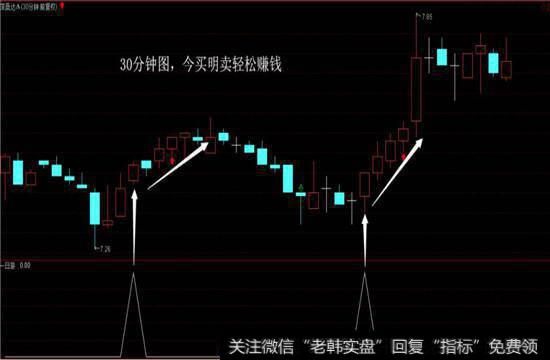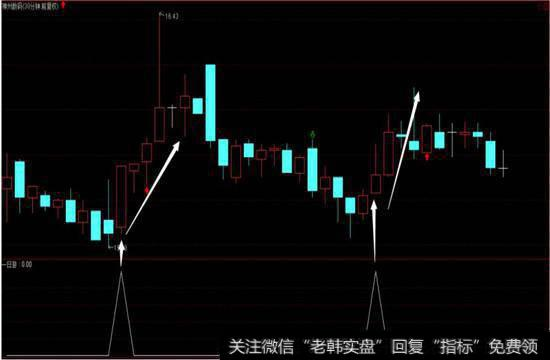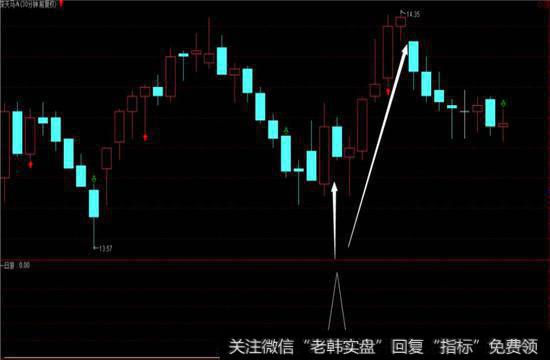1、公式说明：

2、使用周期：

3、操作原理：

1、原理非常简单，就是大家常用的经典CCI指标，平时我们用日周期使用得比较多，在这里用30分钟周期来使用。

2、选出个股后，最好配合尾盘30分钟周期的CCI指标由远离-100的最负的最远端向上运动，当快接近-100时候，就是你抢进去的时候。在你进去后，第二天的股价在剩下的8个30分钟周期向上跑的概率极大，这8个30分钟周期就是出货时机。

3、在大盘大跳水的情况下最好别适用该法，正确性会大大降低。

4、在大盘小跌、盘整、小升、猴市、牛市等情况下，该法皆可使用，而且该法在大盘小跌、盘整、小升、猴市、牛市等情况下的准确率会达到90%以上。

5、除了大盘跳水以外，其他情况下尾盘买进，第二天卖出，成功率极高，第二天几乎稳赚，我很多次买到第二天涨到3-6%的股票，偶尔也会买到第二天是涨停板的股票。再次提示，记得是尾盘30分钟选股操作哦，就算万一失误，第二天也能及时止损出货。

TYP:=(HIGH+LOW+CLOSE)/3;

CCI:=(TYP-MA(TYP,14))/(0.015*AVEDEV(TYP,14));

CCI修正1:=EMA(EMA(EMA(CCI,2),2),2);

CCI修正2:=EMA(EMA(EMA(CCI,3),2),2);

X:=(CROSS(CCI修正1,CCI修正2) OR (CCI修正1 >=CCI修正2))

AND CCI修正1>=-101 AND CCI修正1>=REF(CCI修正1,1)

AND REF(CCI修正1,1)<-101 ;

RSV:=(CLOSE-LLV(LOW,9))/(HHV(HIGH,9)-LLV(LOW,9))*100;

K:=SMA(RSV,3,1);

D:=SMA(K,3,1);

J:=3*K-2*D;

K1:= EMA(EMA(EMA(K,2),2),2);

D1:= EMA(EMA(EMA(D,2),2),2);

J1:= EMA(EMA(EMA(J,2),2),2);

Y:= J1 > REF(J1,1) ;

X AND Y;

VUR0:=(2*O+H+L+3*C)/7;

VUR1:=CAPITAL*VUR0;

VUR2:=SMA(AMOUNT,10,1)/1000;

VUR3:=MA(C*3,5);

VUR4:=VUR2*C*3/VUR3*1/10;

VUR5:=VUR2*O*3/VUR3*1/10;

VUR6:=VUR2*H*3/VUR3*1/10;

VUR7:=VUR2*L*3/VUR3*1/10;

VUR8:=(2*VUR5+VUR6+VUR7+3*VUR4)/7;

VUR9:=DMA(EMA(VUR4,2),SUM(V,1)*VUR8);

VUR10:=EMA(VUR9,2);

VURA:=VUR9>VUR10;

VURB:=EMA(VUR1-VUR9,1);

VURC:=REF(VURB,1);

VURD:=VURB>VURC;

VURS:=VURD AND C>O;

VURE:=(VURB-VURC)*15;

VURF:=(VUR9-VUR10)*15000;

VURG:=(VURC-VURB)*(-1);

VURH:=IF(VURB>VURC,VURE/1000,0);

VURN:=IF(VURB

VURT:=IF(VURA AND VURS,VURF/10000,0);

VURL:=IF(VURA AND VURB

STICKLINE(VURB>VURC,0,VURE,3,1),COLORRED;

STICKLINE(VURB

STICKLINE(VURA AND VURS,0,VURF,3,0),COLORYELLOW;

STICKLINE(VURA AND VURB